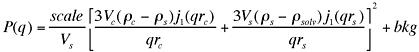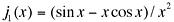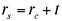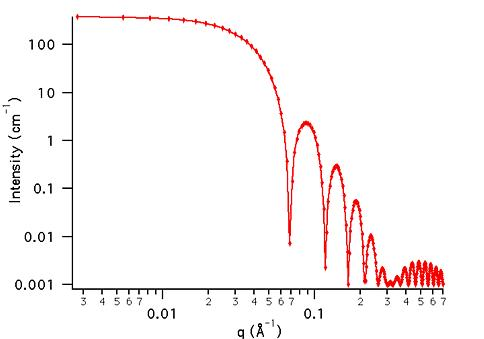MODEL

CoreShell

ORIGINAL AUTHOR/MODIFICATION

Steve Kline 06 NOV 1998

Alan Munter 08 JULY 1999, converted to Java

APPROVED FOR DISTRIBUTION

DESCRIPTION

Calculates the form factor, P(q), for a monodisperse spherical particle with a core-shell structure. The form factor is normalized by the total particle volume.

Resolution smeared version is also provided.

VARIABLES

Input Variables (default values):

Parameter Variable Value
0Scale1.0
2Shell Thickness (Å)10.0
3Core SLD (Å-2)1.0e-6
4Shell SLD (Å-2)2.0e-6
5Solvent SLD (Å-2)3.0e-6
6incoherent Background(cm-1)0.000

USAGE NOTES

The function calculated is:where,, and. The returned value is scaled to units of [cm-1].Parameter (scale) and the SLD's are multiplicative factors in the model and are perfectly correlated. No more than one of these parameters can be free during the model fitting.

If the scale factor Parameter is set equal to the particle volume fraction, phi, the returned value is the scattered intensity per unit volume, I(q) = phi*P(q). However, no interparticle interference effects are included in this calculation.

Scattering from monodisperse vesicles can be calculated by setting the core SLD equal to the solvent SLD.

REFERENCE

Guinier, A. and G. Fournet, "Small-Angle Scattering of X-Rays", John Wiley and Sons, New York, (1955).

TEST DATASET

This example dataset is produced by calculating the CoreShell, using 256 data points, qmin = 0.001 Å-1, qmax = 0.7 Å-1 and the above default coefficient values.